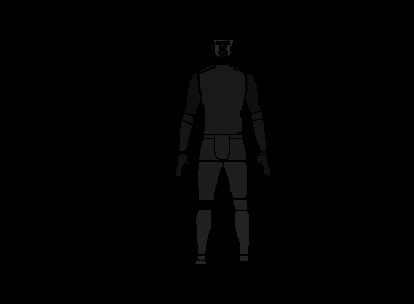# ValueError: All bounding boxes should have positive height and width. Found invalid box [790.0323486328125, 359.0328369140625, 790.0323486328125, 359.0328369140625] for target at index 0

Can any one help with this issues. Searched the internet to see if I could resolve but not yet. Will appreciate any advice.

I am following the transfer learning fine turning tutorial which can be found here on pytorch site: https://pytorch.org/tutorials/beginner/transfer_learning_tutorial.html

I am how ever training with 39 classes.

Here is my code for the dataset:

``````class Dataset(torch.utils.data.Dataset):
def __init__(self, root, transforms=None):
self.root = root
self.transforms = transforms
# load all image files, sorting them to
# ensure that they are aligned
self.imgs = list(sorted(os.listdir(os.path.join(root, "seg_image_use"))))

def __getitem__(self, idx):
img_path = os.path.join(self.root, "seg_image_use", self.imgs[idx])
img = Image.open(img_path).convert("RGB")
# note that we haven't converted the mask to RGB,
# because each color corresponds to a different instance
# with 0 being background

# instances are encoded as different colors
obj_ids = np.unique(mask)[1:] # first id is the background, so remove it
# get bounding box coordinates for each mask
num_objs = len(obj_ids)
boxes = []
for i in range(num_objs):
xmin = np.min(pos)
xmax = np.max(pos)
ymin = np.min(pos)
ymax = np.max(pos)
boxes.append([xmin, ymin, xmax, ymax])

# convert everything into torch.Tensor
boxes = torch.as_tensor(boxes, dtype=torch.float32)
area = (boxes[:, 3] - boxes[:, 1]) * (boxes[:, 2] - boxes[:, 0])

target = {}
target["boxes"] = boxes
target["labels"] = torch.as_tensor(obj_ids, dtype=torch.int64) - 1 # corrected by Rawi
target["image_id"] = torch.tensor([idx])
target["area"] = area
target["iscrowd"] = torch.zeros((num_objs,), dtype=torch.int64) # suppose all instances are not crowd

if self.transforms is not None:
img, target = self.transforms(img, target)

return img, target

def __len__(self):
return len(self.imgs)
``````

Here is the error I get:

``````---------------------------------------------------------------------------
ValueError                                Traceback (most recent call last)
<ipython-input-57-c798930961c1> in <module>
4 for epoch in range(num_epochs):
5     # train for one epoch, printing every 10 iterations
----> 6     train_one_epoch(model, optimizer, data_loader, device, epoch, print_freq=10)
7     # update the learning rate
8     lr_scheduler.step()

~\measurement_model_dev\engine.py in train_one_epoch(model, optimizer, data_loader, device, epoch, print_freq)
28         targets = [{k: v.to(device) for k, v in t.items()} for t in targets]
29
---> 30         loss_dict = model(images, targets)
31
32         losses = sum(loss for loss in loss_dict.values())

~\anaconda3\envs\measurement_py37\lib\site-packages\torch\nn\modules\module.py in _call_impl(self, *input, **kwargs)
725             result = self._slow_forward(*input, **kwargs)
726         else:
--> 727             result = self.forward(*input, **kwargs)
728         for hook in itertools.chain(
729                 _global_forward_hooks.values(),

~\anaconda3\envs\measurement_py37\lib\site-packages\torchvision\models\detection\generalized_rcnn.py in forward(self, images, targets)
92                     raise ValueError("All bounding boxes should have positive height and width."
93                                      " Found invalid box {} for target at index {}."
---> 94                                      .format(degen_bb, target_idx))
95
96         features = self.backbone(images.tensors)

ValueError: All bounding boxes should have positive height and width. Found invalid box [790.0323486328125, 359.0328369140625, 790.0323486328125, 359.0328369140625] for target at index 0.
``````

I’m not sure what data you are using, so I can’t say for sure, but it looks like your problem is with how you are loading your bounding boxes in the dataset. The error shows that the bounding box is [790.0323486328125, 359.0328369140625, 790.0323486328125, 359.0328369140625], so the xmin and xmax are equal and the ymin and ymax are equal, resulting in a 0x0 box.

My hunch would be that the issue lies in how you are getting your dimensions in this part, but I don’t know what your annotations look like, so I can’t say for sure.

``````xmin = np.min(pos)
xmax = np.max(pos)
ymin = np.min(pos)
ymax = np.max(pos)
``````

I agree with you, I am not just sure what to do. I am going to include my annotation here. I am using the Image(.jpg) and Mask(.png). The annotation has 38 classes + background.The Process I used to Prepare my Custom Datatset:

1. I annotated body parts of interests with lableme annotation tool.
2. I then generated a mask image with colors to indicate the parts of interests.(as seen in the colored image I uploaded)